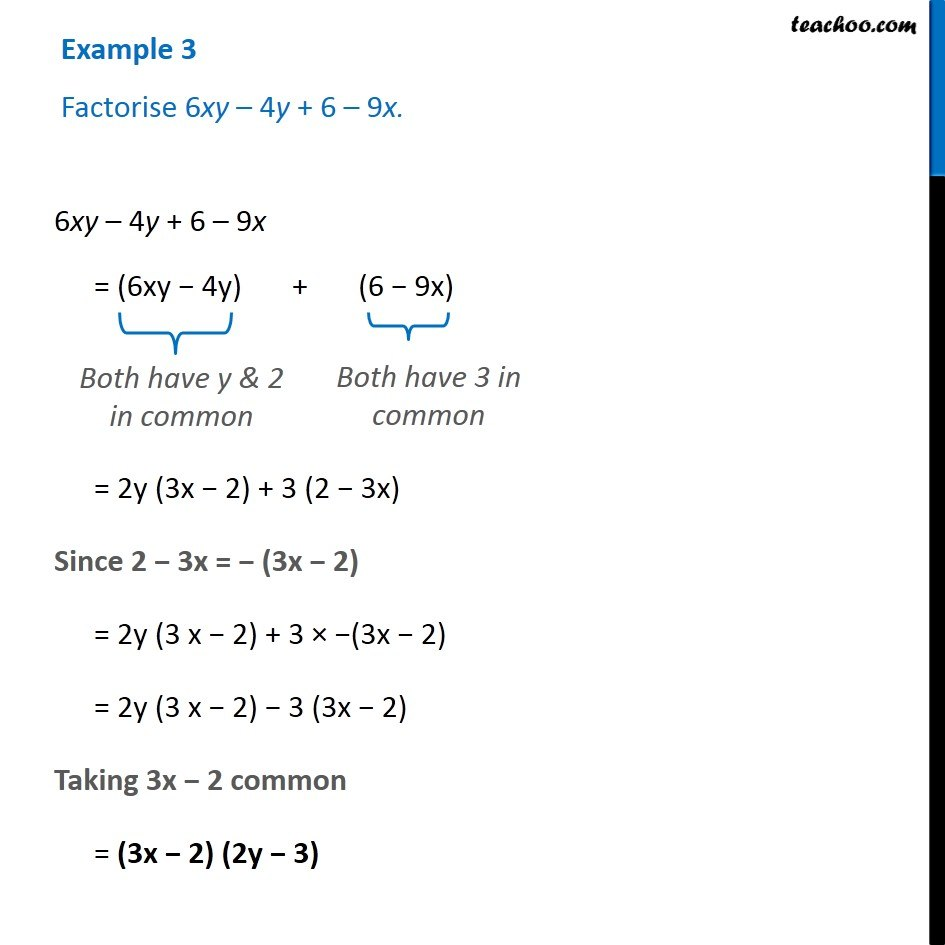Examples

Chapter 14 Class 8 Factorisation
Serial order wise### Transcript

Example 3 Factorise 6xy – 4y + 6 – 9x. 6xy – 4y + 6 – 9x = (6xy − 4y) + (6 − 9x) Both have y & 2 in common Both have 3 in common = 2y (3x − 2) + 3 (2 − 3x) Since 2 − 3x = − (3x − 2) = 2y (3 x − 2) + 3 × −(3x − 2) = 2y (3 x − 2) − 3 (3x − 2) Taking 3x − 2 common = (3x − 2) (2y − 3)